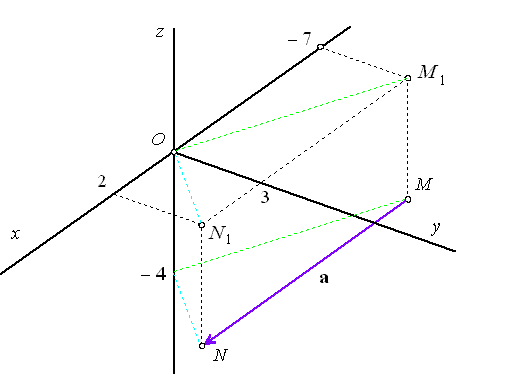Example 7. From Cartesian coordinates find homogeneous coordinates of real point $M=\left(-7,3,-4\right)$ and ideal point ${}_{\infty }U$ determined by direction of vector $\mathbf{a}=MN,\phantom{\rule{2mm}{2mm}}N=\left(2,3,-4\right).$

Solution. Homogeneous coordinates of the real point M form a class of equivalent quadruples

 $M=k.\left(-7,\phantom{\rule{2mm}{2mm}}3,\phantom{\rule{2mm}{2mm}}-4,1\right),\phantom{\rule{2mm}{2mm}}k\ne 0$

where the last coordinate ${a}_{0}=1$ in the normal form.

Homogeneous coordinates of the ideal point ${}_{\infty }U$ are defined from the coordinates of the endpoint M and N of direction vector $\mathbf{a}$ in the normal form

 $\mathbf{a}=\stackrel{\to }{MN}=N-M=\left(2,3,-4,1\right)-\left(-7,3,-4,1\right)=\left(9,0,0,0\right)$

and they form a class of equivalent quadruples

 ${}_{\infty }U=k.\left(9,0,0,0\right),\phantom{\rule{2mm}{2mm}}k\ne 0$Figure 1: Homogeneous coordinates